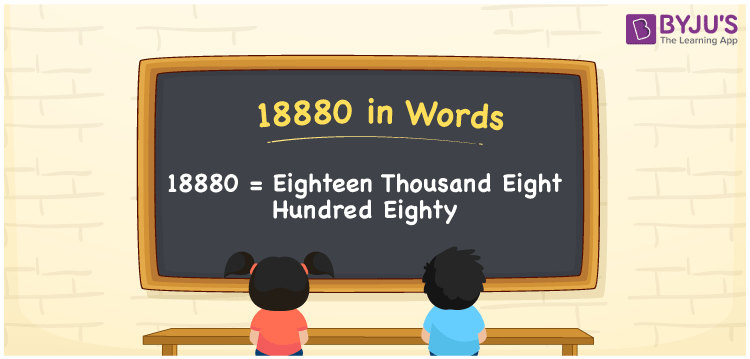# 18800 in Words

18800 in words can be written as Eighteen Thousand Eight Hundred. The article of 18800 in words will provide students with a clear knowledge of the concept of counting numbers. If Rs. 18800 is spent on buying new clothes, then you can say that “I spent Eighteen Thousand Eight Hundred Rupees in buying new clothes”. The  concept of converting 18800 into words using the English alphabet is discussed here. Students will be able to convert numbers in words with the help of the resources available at BYJU’S. 18800 can be read as “Eighteen Thousand Eight Hundred” in English.

 18800 in words Eighteen Thousand Eight Hundred Eighteen Thousand Eight Hundred in Numbers 18800

## 18800 in English Words## How to Write 18800 in Words?

In this section you will learn in detail about conversion of 18800 into words using the place value chart. Five digits are present in 18800. The place value chart of 18800 can be understood easily using the table below.

 Ten Thousands Thousands Hundreds Tens Ones 1 8 8 0 0

The expanded form of the number 18800 is given below:

1 x Ten Thousand + 8 × Thousand + 8 × Hundred + 0 × Ten + 0 × One

= 1 x 10000 + 8 × 1000  + 8 × 100 + 0 × 10 + 0 × 1

= 10000 + 8000 + 800

= 18800

= Eighteen Thousand Eight Hundred

Hence, 18800 in words is written as Eighteen Thousand Eight Hundred.

18800 is a natural number that precedes 18801 and succeeds 18799.

18800 in words – Eighteen Thousand Eight Hundred

Is 18800 an odd number? – No

Is 18800 an even number? – Yes

Is 18800 a perfect square number? – No

Is 18800 a perfect cube number? – No

Is 18800 a prime number? – No

Is 18800 a composite number? – Yes

## Frequently Asked Questions on 18800 in Words

Q1

### Write 18800 in words.

18800 can be written as “Eighteen Thousand Eight Hundred” in words.
Q2

### Is 18800 an odd or even number?

18800 is an even number because it is divisible by 2.
18800/2 = 9400
Q3

### Write Eighteen Thousand Eight Hundred in numbers.

Eighteen Thousand Eight Hundred can be written in numbers as 18800.